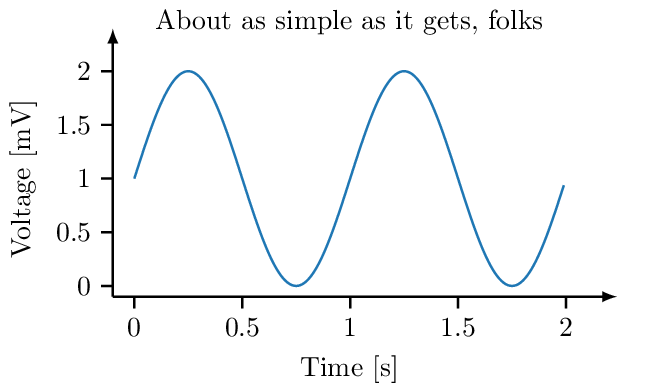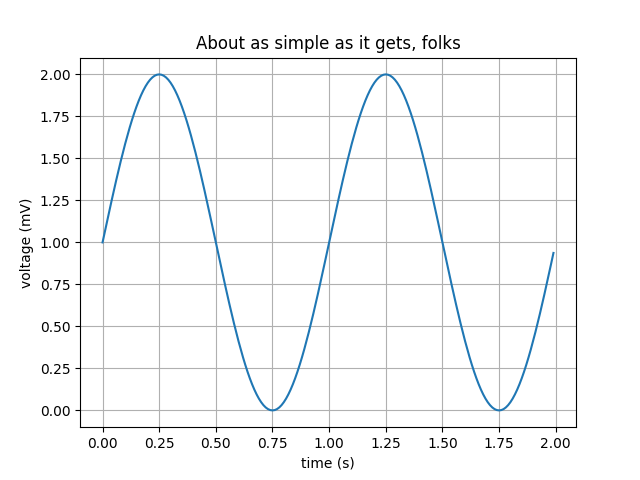Martin Isaksson

### Tags

Figures in scientific papers often look less than professional, and sometimes this can even get in the way of understanding the figure. In this blog post we show how to use matplotlib and tikzplotlib to make publication ready figures that look great and can be styled from the document preamble. Beautiful and understandable figures can possibly lead to higher publication acceptance rate, at least I hope so…

## Introduction

For generating figures in my scientific papers I use TikZ (missing reference) but until recently I have been using matplotlib (missing reference) for graphs and saved them as PDF. When I found tikzplotlib (missing reference) I found it to be awesome but needing a few tweaks. tikzplotlib is a Python tool for converting matplotlib figures into PGFPlots (Feuersänger, 2014) figures for inclusion into LaTeX documents.

If there’s one thing I loath (and you should too), it’s chartjunk (missing reference). We should avoid chartjunk at all cost. Chartjunk is everything in your figure that dilutes your message and adds confusion (missing reference). Before tikzplotlib, I spent a lot of time styling individual plots with matplotlib. With tikzplotlib we can put most of that styling in the LaTeX document header.Example figure produced with this method.

In this blog post we will explore matplotlib and tikzplotlib, remove some chartjunk and build a pipeline to include matplotlib images in LaTeX figures.

## Using tikzplotlib

### Making a plot

The first thing we need to do is to make a plot. We’ll look at the simple plot example from the matplotlib gallery (missing reference). In my opinion this example has the appearance of a matplotlib plot, and I’d like it to look a little more professional.Example figure from the Matplotlib gallery.

At the end we are importing tikzplotlib and using the save function to save a tikz file containing the plot.

For this example, we are using the following packages.

Now we are ready to include this file in a document. Here we are using a TikZ standalone-document as an example to include the tikz code in the document, but some publishers rather want you to include a PDF-version.

We can compile this as a standalone figure into PNG and PDF by running pdflatex -shell-escape figure.tex.

### Tweaking the output

If we look at the LaTeX code above, we see pgplotset with some options. These options are used to set a fixed size of the plot area (excluding the axes labels and the title), set the number of tickmarks etc. We are also redefining the line width semithick to be as thick as thick.

The bounding box of figures produced in this way can be slightly different depending on the labels and title and other things drawn outside the plot area. This is usually not a problem, but will be painfully obvious if two figures are placed side-by-side. Therefore, we need to manually set the bounding box. We do this with a sed hack that inserts a path on line 3 in the file figure.pgf.

This will add an invisible rectangle outside the figure, which will be used by TikZ to determine the bounding box. It will be the same for every figure we make, and in this way we can make sure that two figures side by side will line up perfectly.

### Automation

To automate this build, we can use the following Makefile. The input files are figure.py and figure.tex, both of which are listed above.

We want to create the file figure.png. To do this we start with running Python to generate the .pgf file that we then include in figure.tex. Compiling figure.tex renders the figure and saves it as a .png. We get a .pdf for free when using pdflatex.

### Including the figure in a document

We need to include the produced figure into a figure environment in our document, and for this we can write the LaTeX code below (missing reference).

If your publisher requires you to submit a manuscript with PDF figures, you can use the standalone document above and use the standard \includegraphics command instead of the \input command.

(missing reference) listed ten simple rules for better figures, of which rule number 5 was Do not trust the default and number 8 was related to avoiding chartjunk. It also listed a few good tools, among others matplotlib, TikZ and PGF, but didn’t tell us how to use them.

The entire library of work by Edward Tufte is hugely inspirational to us. (missing reference) tells us not to put too much ink on the paper and gives us examples on how to achieve this.

(missing reference) teaches us how to use TikZ for non-plots. Besides being a good introduction to TikZ we also learn how to compile standalone pictures and how to embed the TikZ code into a LaTeX figure.

## Conclusion

We have looked at how to make matplotlib figures look more professional by using tikzplotlib and some tweaks. The Makefile we created should go into the figures directory of your manuscript so that you can use make -C figures all as a dependency to your normal make report target.

I find it easy to control the style of my plots from the preamble of my manuscript and just rebuild the plots if the content changed.

In my humble opinion, easily understandable figures that makes it easy for a reviewer to understand the message that your are trying to send, should make it harder for the reviewer to reject your paper. In fact there is some evidence (Huang, 2018) that the visual appearance of a paper is important and that improving the paper gestalt reduces risk of getting rejected.

1. Feuersänger, C. (2014). Manual for Plotting Package pgfplots. 1–500. https://ctan.org/pkg/pgfplots
2. Huang, J.-B. (2018). Deep Paper Gestalt. CoRR, abs/1812.0. http://arxiv.org/abs/1812.08775

## Suggested citation

If you would like to cite this work, here is a suggested citation in BibTeX format.